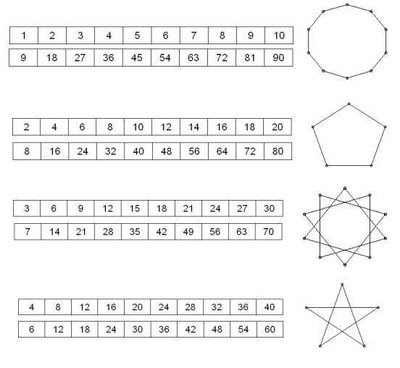## Monday, June 8, 2009

### Polygons and the Multiplication TableI was inspired to look at star polygons like the one above this after seeing this post by David Vancouvering, which was picked up by math teachers at play. It seems that there are a number of ways to use these polygon diagrams to explore multiplication and division facts.

Basically, students explore patterns in the digits of the multiplication table in the following way:1. choose a row in the 10x10 multiplication table (for example, the row that shows the 6 times table);
2. put your pencil at "0" on the 10-point circle diagram;
3. looking only at the last digit of each entry in the row, draw a line to the point that has that digit (draw a line from 0 to 6);
4. continue until you have drawn a line back to zero (draw lines 6 to 2, 2 to 8, 8 to 4, 4 to 0).

Another way to generate the same diagram is to:

1. pick a number n (say 6);
2. put your pencil at "0" on the 10-point circle diagram;
3. moving clockwise, skip-count by n (skip over n-1 dots - in this example, skip over 5 dots);
4. draw connecting lines between the dots you land on (in order) until you reach zero (draw lines 0 to 6, 6 to 2, 2 to 8, 8 to 4, 4 to 0). The numbers you land on will match the last digits of the n times table (in order).

These diagrams were likely much more common in the 'new math' curriculum of the 60s and 70s when modular arithmetic became a standard part of grade-school mathematics. Here, in looking at the last digit of a number, we are evaluating the multiples "mod 10" or, in the language that was used in the (old) new math, we are doing "clock arithmetic on a 10-hour clock."This highlights a nice symmetry among the last digit of the n and 10-n multiplication tables.